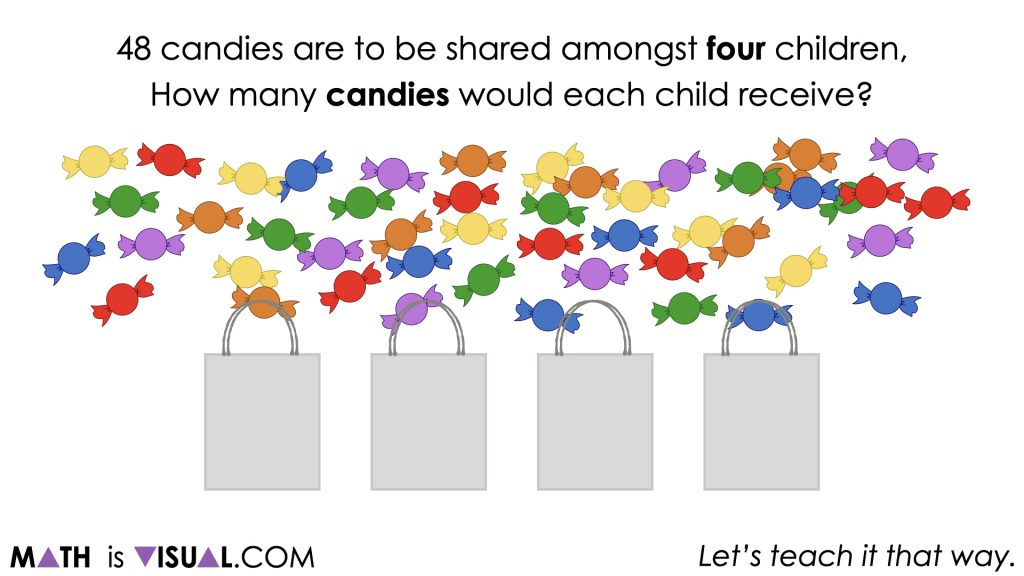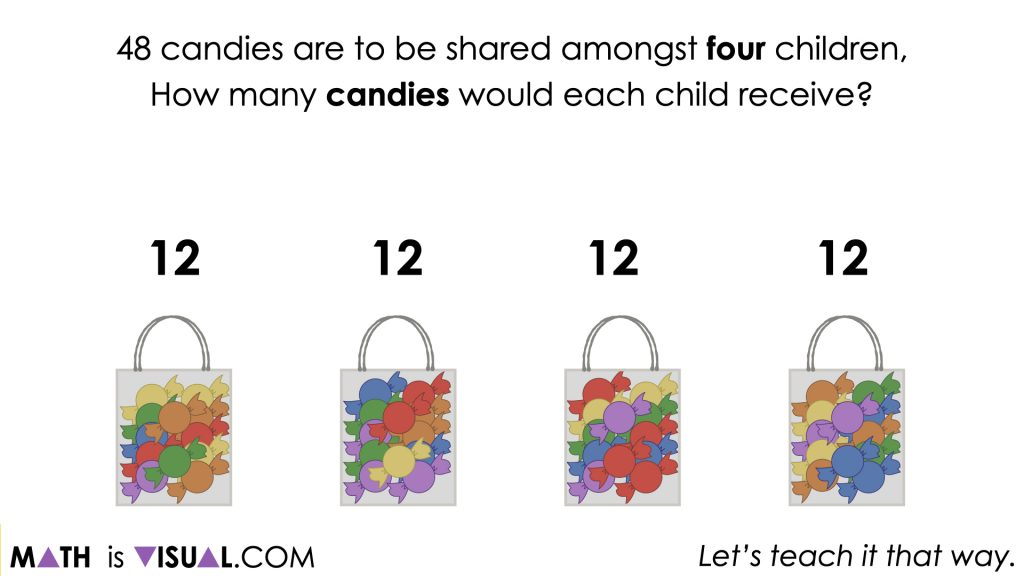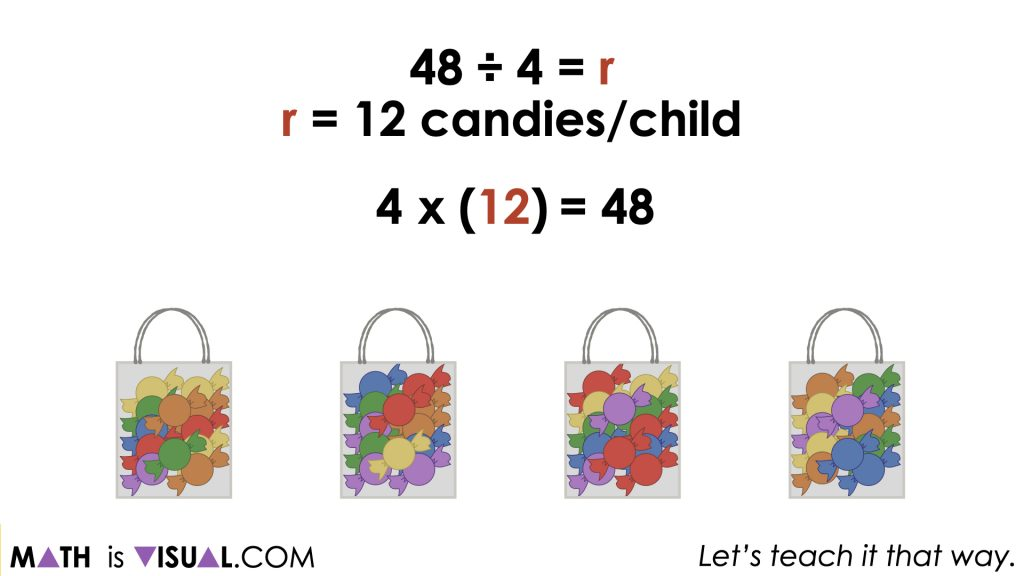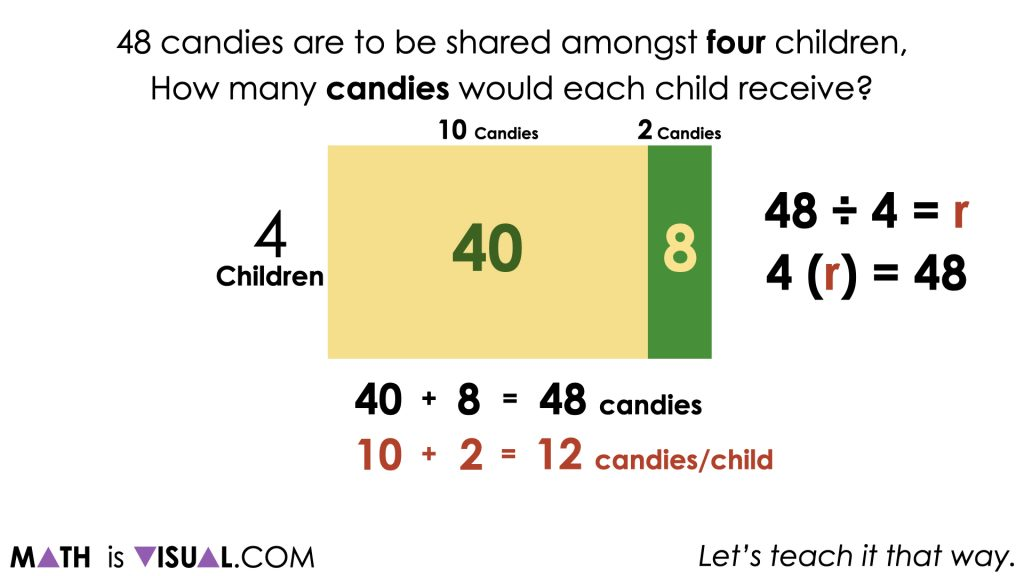# Using Division to Solve One-Step Equations

Visual math talk prompts highlighting the relationship between multiplication and division to solve one-step algebraic equations.

## In This Set of Math Visual Prompts…

Students will explore partitive division problems with context and connect them to one-step algebraic equations.

## Intentionality…

This set of visual math talk prompts is taken from the Math Talk section of Day 2 in the Make Math Moments Problem Based Unit called Sowing Seeds Revisited. The purpose of the Math Talk is to reinforce key concepts and big ideas from this problem based math unit including:

• There are two types of division;
• Partitive division is when the total quota is known (the dividend), and the number of parts or groups (the divisor) is known;
• Partitive division reveals a rate;
• In partitive division, the dividend and the divisor often have different units;
• The dividend from any division sentence can be decomposed into smaller parts to allow for friendlier division by the divisor. This strategy is known as partial quotients. (i.e.: 85 ÷ 5 = 45 ÷ 5 + 40 ÷ 5 = 9 + 8 = 17);
• Division is the inverse operation of multiplication;
• Variables are used to represent changing or unknown quantities;
• When solving an equation where the coefficient of the unknown variable is not equal to 1, division is required to determine the value of the unknown variable and the context of the problem determines which type of division is required.

Unsure about some of the terminology listed above?

## Preparing to Facilitate

Present the following multiplication and division equations one at a time. Encourage students to describe a partitive context that could be represented by each of the division sentences such as seeds (dividend) and planting pots (divisor) like we did in the last lesson.

Students should use a model of their choice to solve and/or represent their thinking.

48 ÷ 4 = r

4(r) = 48

Two (2) additional prompts are shared in the Teacher Guide from Day 2 of the Sowing Seeds Revisited problem based math unit

Consider leveraging the silent solution animations below to help you prepare to facilitate this math talk. The purpose of this math talk is to reveal that both the division and one-step algebraic equation can be solved using the same strategy and model.

## Problem #1:

In this visual math talk prompt we begin with a low floor partitive division problem asking students:

48 candies are to be shared amongst four children.

How many candies would each child receive?A natural strategy that suits this context and type of division is fair sharing. The candies can be partitioned into four bags equally by fair sharing one-by-one, two-by-two, ten-by-ten or however students choose to unitize for sharing across the bags.From here, we can ask students what operation they utilized for this problem. While the context is sending a clear call for partitive division, some students might think to the strategy they leveraged (i.e.: skip counting) and believe it was addition or even subtraction as they “removed” candies from the total to distribute to the bags. It is helpful to highlight that while this is a partitive division problem, multiplication can be used as a strategy to solve the problem.To highlight how division and multiplication are intrinsically related, we can also leverage an area model to highlight how a single model can be used to solve multiplication and division problems.Therefore, there is 2 thirds of 120 metres in 80 metres.

## Want to Explore These Concepts & Skills Further?

Four (4) additional math talk prompts are available in Day 2 of the Sowing Seeds Revisited problem based math unit that you can dive into now.

Why not start from the beginning of this contextual 5-day unit of ratios and rates real world lessons from the Make Math Moments Problem Based Units page.

Did you use this in your classroom or at home? How’d it go? Post in the comments!

Math IS Visual. Let’s teach it that way.